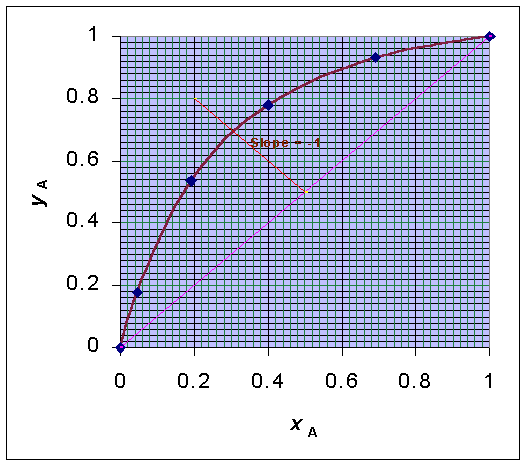### Flash Distillation

A feed of 50 mole % hexane and 50 mole % octane is fed into a pipe still through a pressure reducing valve and then into a flash disengaging chamber. The vapor and liquid leaving the chamber are assumed to be in equilibrium. If the fraction of the feed converted to the vapor is 0.5, find the compositions of the top and bottom products. The following table gives the equilibrium data for this system.

 Mole fractions of hexane in liquid x 1 0.69 0.4 0.192 0.045 0 Mole fractions of hexane in vapor y 1 0.932 0.78 0.538 0.1775 0

Calculations:

Basis: 100 mole of feed

F = 100 mol

zF = 0.5

D = 100 x 0.5 = 50 mol

W = F - D = 50 mol

Slope of the operating line = -W/D = -1

With these data, the equilibrium curve and operating line for flash distillation are plotted as follows:From the plot, it could be seen that the operating line cuts the equilibrium curve at the point (0.31, 0.69). That is yD = 0.69 and xW = 0.31

Composition of top product = yD = 69% Hexane

Composition of bottom product = xW = 31% Hexane